# Resistor applications

Resistors are used in a wide variety of applications. This category contains pages explaining various resistor applications.

## Resistors in Series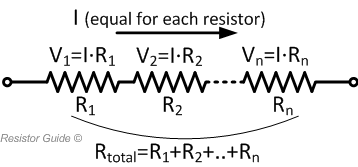In series connection of resistors, current through them is equal to each member as there is only one path for the current to flow. The voltage drop is proportional to the resistance of each individual resistor. The total resistance of the connection is represented as: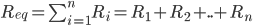## Resistors in ParallelIn parallel connection of resistors, the voltage across resistor is equal for each member. The current however, is proportional to the resistance of each resistor. Total resistance is represented as: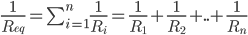## Heating Resistor

Heater resistors are used when an electronic device needs to generate heat. They are specially designed types of resistors that can provide a reliable and controllable heat.

A heating resistor can create a convective heat, as it heats up the surrounding air, or radiant heat, making it heat up other objects through infrared radiation. Radiant heating oftentimes requires the heater resistor to be placed within the line of sight of the object that is to be heated, with the help of a fan to blow over heater resistors to increase the heating effectiveness.

## Current Limiting Resistor

A current limiting resistor is a resistor used to reduce the current present in a circuit. A simple example is a resistor in series with a LED. You would usually want to have a current limiting resistor in series with the LED to control the amount of current flowing through it, else the LED will burn due to it exceeding its current limit.

To calculate the resistance value of the resistor to be used, it is represented by: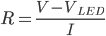Where V is the source voltage VLED is the LED voltage and I is the LED current.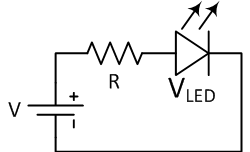## Pull-up / Pull-down Resistor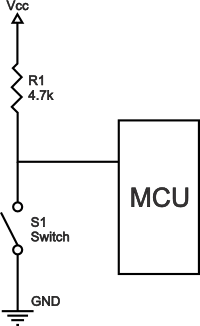Pull-up resistors are resistors commonly used in logic circuits to help ensure a well-defined logical level at a pin under all conditions. It is used to prevent the logic state to be left as “floating. The pull-up resistor solves this problem by pulling the value to a logical high state when switch S1 is open instead of leaving it floating.

Pull-up resistors are not a special kind of resistors, they are simple fixed-value resistors connected between the voltage supply and the appropriate pin, which results in defining the input or output voltage in the absence of a driving signal.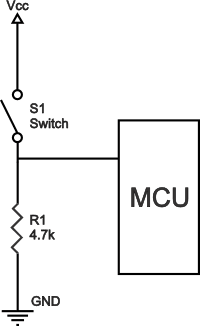Pull-down resistors work in a similar manner as a pull-up resistor, except that they pull the pin to a logical low value. They are connected between ground and an appropriate pin on the device.

An example of a pull-down resistor used in a digital circuit can be seen in the figure above. A pushbutton switch S1 is connected between the supply voltage and a microcontroller pin. When the switch is closed in the said circuit, the microcontroller input is at a logical high value, but when the switch is open, the pull-down resistor will pull the input voltage down to ground (logical zero), preventing an undefined state.

Pull-down resistors must have a larger resistance than the impedance of the logic circuit, or it might pull the voltage down too much making the input voltage at the pin remain at a constant logical low value, with no regard to the switch position.